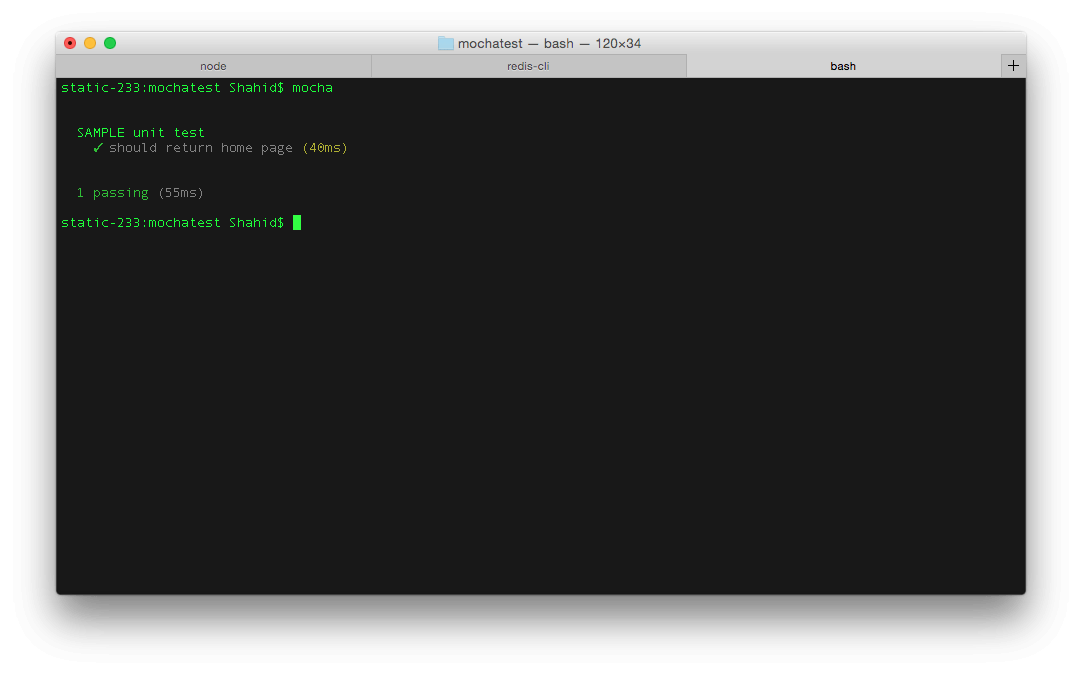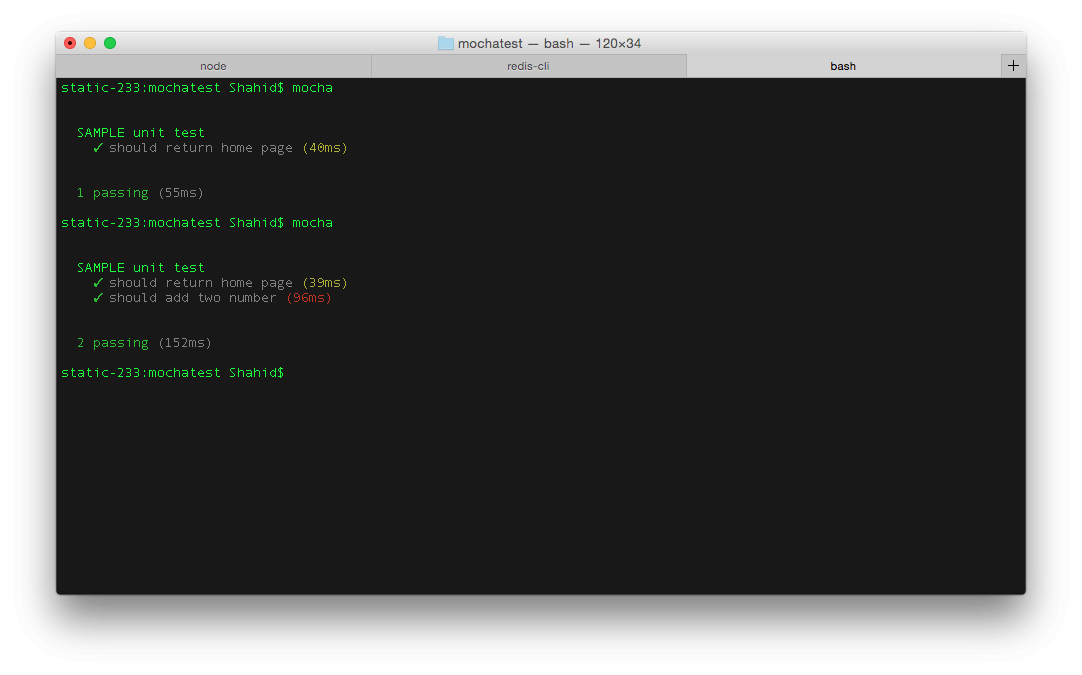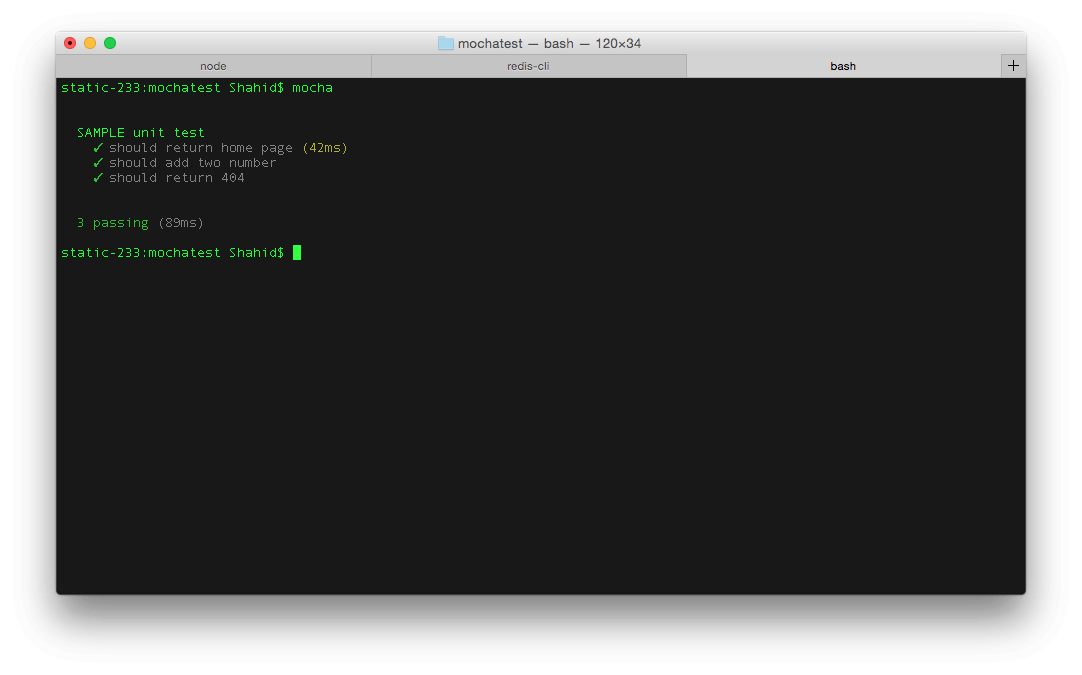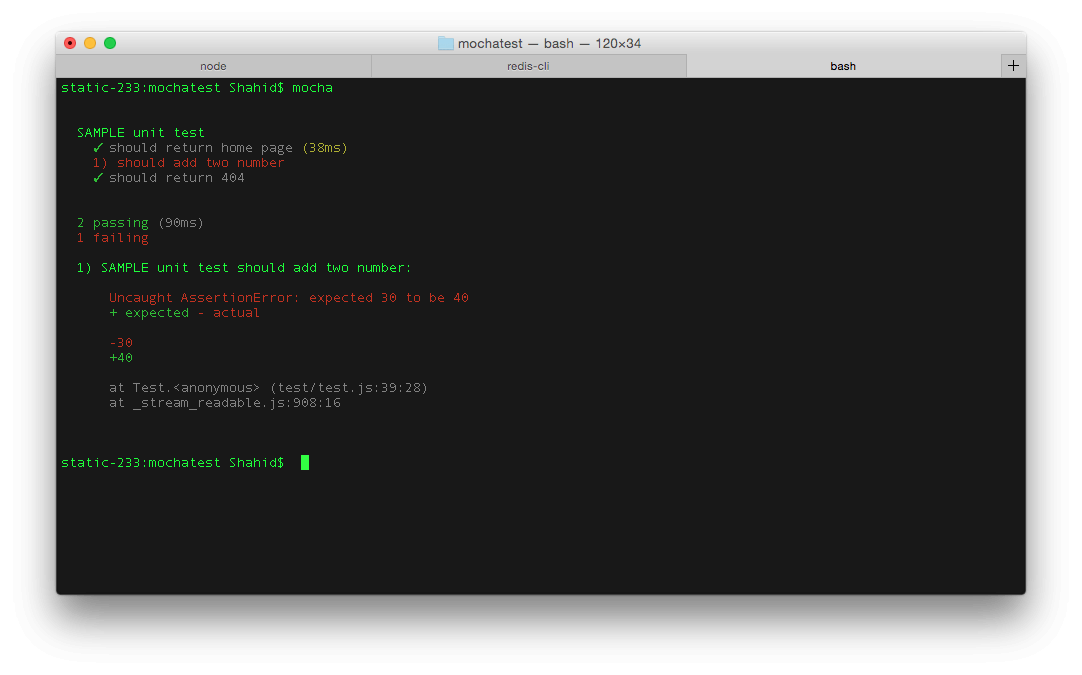# How to Test Nodejs Code and RESTful API

One of the important task which most of the developers ignores ( i used to ignore too ) is writing unit tests for your code. Unit test is not just a help to tester or quality assurance team but its the proof of quality of your code.

In this tutorial i am going to cover how to test your REST api’s written in ExpresJS using famous unit testing tool called Mocha and supertest.

### What is Mocha ?

Mocha is simple and easy to use test framework. It runs on Node.js and allows you to develop test cases for your program and execute them in serial with proper reporting.

To use mocha i suggest you to install it and as global package using following command.

sudo npm install -g mocha

### What is supertest ?

Supertest is library written to test HTTP calls in node.js. So if you want to write test cases which going to do some HTTP calls ( GET, POST, PUT etc ) then this might be the useful tool for you.

## Our project :

Let’s develop an expressJS app with some routes and test whether those are giving expected result or not.

Here is package.json :

{
"name": "MochaTest",
"version": "0.0.1",
"dependencies": {
"body-parser": "^1.13.2",
"express": "^4.13.1"
}
}

Install dependencies by running

npm install

on terminal.

Here is Server file.

var express = require("express");
var bodyParser = require("body-parser");
var app = express();
app.use(bodyParser.urlencoded());
app.use(bodyParser.json());
var router = express.Router();

router.get('/',function(req,res){
res.json({"error" : false, "message" : "Hello !"});
});

res.json({"error" : false, "message" : "success", "data" : req.body.num1 + req.body.num2});
});

app.use('/',router);

app.listen(3000,function(){
console.log("I am listening at PORT 3000");
})

## Writing test cases :

Let’s move ahead and write some unit test for above program. First you need to install mocha, should and supertest in your project. It’s better if we add them in dev dependencies.

1 : Install mocha.

npm install --save-dev mocha

2 : Install should.

npm install --save-dev should

3 : Install supertest.

npm install --save-dev supertest

After running above commands your package.json should look this.

{
"name": "MochaTest",
"version": "0.0.1",
"dependencies": {
"body-parser": "^1.13.2",
"express": "^4.13.1"
},
"devDependencies": {
"mocha": "^2.2.5",
"should": "^7.0.2",
"supertest": "^1.0.1"
}
}

Let’s create one folder called “test” and place “test.js” inside it. Just to separate it from rest of the code.

Before writing unit test, let’s discuss how the building block will look.

You need to provide description of unit test using “describe” function and use “it” to define several unit test cases into it. “it” provides us “done” function is used to indicate the end of test case.

Let’s write following unit test case.

• 404 for other router

var supertest = require("supertest");
var should = require("should");

// This agent refers to PORT where program is runninng.

var server = supertest.agent("http://localhost:3000");

// UNIT test begin

describe("SAMPLE unit test",function(){

server
.get("/")
.expect("Content-type",/json/)
.expect(200) // THis is HTTP response
.end(function(err,res){
// HTTP status should be 200
res.status.should.equal(200);
// Error key should be false.
res.body.error.should.equal(false);
done();
});
});

});

Assuming you have installed mocha globally, run your node program and listen on port 3000. Open another tab and run

mocha

to start test.

Here is screenshot.var supertest = require("supertest");
var should = require("should");

// This agent refers to PORT where program is runninng.

var server = supertest.agent("http://localhost:3000");

// UNIT test begin

describe("SAMPLE unit test",function(){

-------------------------------------
});

server
.send({num1 : 10, num2 : 20})
.expect("Content-type",/json/)
.expect(200)
.end(function(err,res){
res.status.should.equal(200);
res.body.error.should.equal(false);
res.body.data.should.equal(30);
done();
});
});

});

Here is test case response.### Test case 3 : 404 Error.

var supertest = require("supertest");
var should = require("should");

// This agent refers to PORT where program is runninng.

var server = supertest.agent("http://localhost:3000");

// UNIT test begin

describe("SAMPLE unit test",function(){

---------------------------------
});

---------------------------------
});

it("should return 404",function(done){
server
.get("/random")
.expect(404)
.end(function(err,res){
res.status.should.equal(404);
done();
});
})
});

Here is the output.### Let’s see some failure case

Just change the equal value in second test to other than 30 and see the response. Like this.

var supertest = require("supertest");
var should = require("should");

// This agent refers to PORT where program is running.

var server = supertest.agent("http://localhost:3000");

// UNIT test begin

describe("SAMPLE unit test",function(){

--------------------------------
});

server
.send({num1 : 10, num2 : 20})
.expect("Content-type",/json/)
.expect(200)
.end(function(err,res){
res.status.should.equal(200);
res.body.error.should.equal(false);
res.body.data.should.equal(40);
done();
});
});

it("should return 404",function(done){
---------------------------
})
});

You should get one failure test case.## Conclusion:

Writing unit test is like never-ending process. You can write almost all kind of combination of test cases. You should learn and start writing test cases right after you finish one particular module,don’t wait for the project to finish.